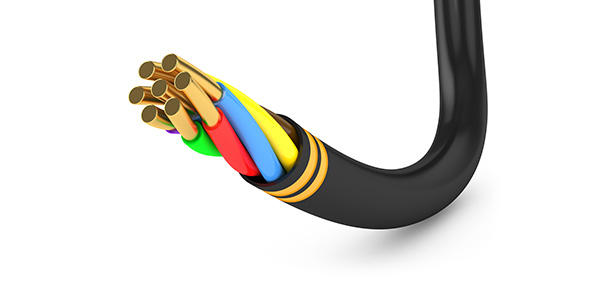# Capacitors, Cables, Bus Bars, Test Lab

41 Questions | Total Attempts: 667SettingsCreate your own QuizThis is your description.

• 1.
What is the effect of series capacitors on a transmission line?
• A.

Shortens the line electrically

• B.

Auxiliary synchronous machines

• C.

Impedance

• D.

Lengthens the line electrically

• 2.
The purpose of adding series capacitors on a transmission line is to
• A.

Increase flow on the line by decreasing impedance (making line electrically shorter)

• B.

Compensate for the vars produced by the line

• C.

To increase the total vars

• D.

To decrease the total vars

• 3.
A dielectric material is mostly a  _____?
• A.

Conductor

• B.

Insulator

• C.

Magnetic field

• D.

Metallic

• 4.
When performing an insulation test on a cable, which test is not commonly performed?
• A.

AC/DC breakdown test

• B.

Doble power factor test

• C.

CT saturation test

• D.

Megger test

• 5.
When performing an insulation resistance test which test is most commonly performed?
• A.

AC/DC breakdown test

• B.

Doble power factor test

• C.

CT saturation test

• D.

Megger test

• 6.
When performing a power factor test to test the dielectric which test is most commonly performed?
• A.

AC/DC breakdown test

• B.

Doble power factor test

• C.

CT saturation test

• D.

Megger test

• 7.
Which test can be used to test insulation but can be harmful particularly to dc test?
• A.

AC/DC breakdown test

• B.

Doble power factor test

• C.

CT saturation test

• D.

Megger test

• 8.
Which vertical run of the following cable needs more support
• A.

Aluminum

• B.

• C.

Copper

• D.

All need the same

• 9.
The purpose of dielectric in cable is for
• A.

Reduce void formed during manufacturing

• B.

Increase emissivity

• C.

Increase electric dissipation factor

• D.

Increase breakdown voltage

• 10.
What type of insulation can not be used on 115KV Cable?
• A.

Paper

• B.

Gas

• C.

Oil

• D.

XLPE

• 11.
Which insulation carries the highest voltage?
• A.

Paper

• B.

Gas

• C.

Oil

• D.

XLPE

• 12.
What is the purpose of the semi-conducting material between the conductor and insulation and between the insulation and shield of a synthetic type underground cable?
• A.

Reduce voids forming during manufacturing

• B.

Increase breakdown voltage

• C.

Increase the electric dissipation factor

• D.

Increase emissivity

• 13.
In sizing conductors, what is least considered of the following
• A.

Voltage drop

• B.

Heating effect due to conductor proximity, conduit enclosure

• C.

Tripping of circuit breaker protective device

• 14.
What will limit utilizing the full capacity of conductor
• A.

Series reactance

• B.

Shunt capacitance

• C.

Line losses

• D.

Voltage drop

• 15.
The greatest effect of a fault on a system is on
• A.

Transient

• B.

Subtransient

• C.

• 16.
Equal area criterion for power stability for initial fault clearing is
• A.

Power versus power angle

• B.

Time versus System Angle

• 17.
Emissivity is the measure of the power radiated by a body relative to that radiated by an ideal black body( idealized material which absorbs all incident energy and reflects none)  at the same temperature
• A.

True

• B.

False

• 18.
The absorptivity of a material is affected primarily by its
• A.

Surface

• B.

Elasticity

• C.

Enthalpy

• D.

Divisity

• 19.
What is the effect of adding a series capacitance to long HV 500KV transmission line:
• A.

Increase angle between the sending end voltage and receiving end voltage

• B.

Line becomes electrically longer

• C.

Offset line inductance for maximum power transfer

• D.

• 20.
Bus Bar design using Copper over aluminum because
• A.

Aluminum has higher surface emissivity

• B.

Copper is more corrosive

• C.

Copper has higher thermal coefficient

• D.

Copper has lower thermal coefficient

• 21.
Which of the following changes would make the least difference in the resistance of a ground rod installation?
• A.

Increasing the spacing from 1 to 3 ft

• B.

Doubling the number of ground rods

• C.

Doubling the diameter of the ground rods

• D.

Increasing the depth of the rods from 4 to 6 feets

• 22.
The most important function of the shield on underground cable operated at 2KV or above is to provide
• A.

A moisture barrier for the cable insulation

• B.

Conductance needed for the charging current

• C.

A tangential pattern of electrical stresses

• D.

A radial pattern of electrical stresses

• 23.
Which cable insulation carries the highest voltage
• A.

Paper

• B.

Gas

• C.

Oil

• D.

Silicon

• 24.
What is not an insulation test?
• A.

AC/DC breakdown test

• B.

Double dower factor

• C.

CT saturation test

• D.

Megger test

• 25.
DC Hipot test of equipment is:
• A.

Diaelctric Test

• B.

Pass/Fail

• C.

Non-destructive

• D.

Diaelctric Test and Pass/Fail

• E.

Diaelctric Test and Non-destructive

• F.

Destructive

• G.

Diaelctric Test and Pass/Fail and Destructive

Related TopicsBack to top
×

Wait!
Here's an interesting quiz for you.